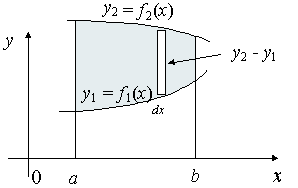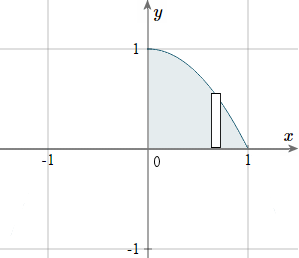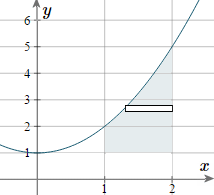Search IntMath
Close

450+ Math Lessons written by Math Professors and Teachers

5 Million+ Students Helped Each Year

1200+ Articles Written by Math Educators and Enthusiasts

Simplifying and Teaching Math for Over 23 Years

# 6. Moments of Inertia by Integration

by M. Bourne

The moment of inertia is a measure of the resistance of a rotating body to a change in motion.

The moment of inertia of a particle of mass m rotating about a particular point is given by:

"Moment of inertia" = md^2

where d is the radius of rotation.

## Inertia for a Collection of Particles

If a group of particles with masses m1, m2, m3, ... , mn is rotating around a point with distances d1, d2, d3, ... dn, (respectively) from the point, then the moment of inertia I is given by:

I = m1d12 + m2d22 + m3d32 +... + mndn2

If we wish to place all the masses at the one point (R units from the point of rotation) then

d1 = d2 = d3 = ... = dn = R and we can write:

I = (m1 + m2 + m3 ... + mn)R2

R is called the radius of gyration.

### Example 1

Find the moment of inertia and the radius of gyration w.r.t. the origin (0,0) of a system which has masses at the points given:

 Mass 6 5 9 2 Point (-3, 0) (-2, 0) (1, 0) (8, 0)

The moment of inertia is:

I = 6(-3)2 + 5(-2)2 + 9(1)2 + 2(8)2

= 54 + 20 + 9 + 128

= 211

To find R, we use:

I = (m1 + m2 + m3 ... + mn)R2

211 = (6 + 5 + 9 + 2)R2

So R ≈ 3.097

This means a mass of 22 units placed at (3.1, 0) would have the same rotational inertia about O as the 4 objects.

Continues below

## Moment of Inertia for AreasWe want to find the moment of inertia, Iy of the given area, which is rotating around the y-axis.

Each "typical" rectangle indicated has width dx and height y2y1, so its area is (y2y1)dx.

If k is the mass per unit area, then each typical rectangle has mass k(y2 y1)dx.

The moment of inertia for each typical rectangle is [k(y2 y1)dx] x2, since each rectangle is x units from the y-axis.

We can add the moments of inertia for all the typical rectangles making up the area using integration:

I_y=kint_a^bx^2(y_2-y_1)dx

Using a similar process that we used for the collection of particles above, the radius of gyration Ry is given by:

R_y=sqrt((I_y)/m

where m is the mass of the area.

### Example 2

For the first quadrant area bounded by the curve

y = 1 − x^2,

find:

a) The moment of inertia w.r.t the y axis. (Iy)

b) The mass of the area

c) Hence, find the radius of gyration

As usual, first we sketch the part of the curve in the first quadrant. It's a parabola, passing through (1, 1) and (0, 1). A "typical" rectangle is shown.In this example,

y_2= 1 − x^2, and y_1= 0, a = 0 and b = 1.

a) Finding Iy:

I_y=k int_a^b x^2(y_2-y_1) dx

=k int_0^1x^2 [(1-x^2)-(0)] dx

=k int_0^1 [x^2-x^4] dx

=k[(x^3)/(3) - (x^5)/(5)]_0^1

=k(1/3-1/5)

= (2k)/15

b) The mass of the area, m.

Now m = kA, where A is the area.

Now

A=int_0^1 (1-x^2) dx

=[x-(x^3)/(3)]_0^1

=1-1/3

 = 2/3

So m = kA = (2k)/3

R_y=sqrt{(I_y)/(m)}

=sqrt{(2k//15)/(2k//3)}

=sqrt[1/5]

 approx 0.447

This means that if a mass of (2k)/3 was placed 0.447 units from the y-axis, this would have the same moment of inertia as the original shape.

For rotation about the x-axis, the moment of inertia formulae become:

I_x=kint_c^(d)y^2(x_2-x_1)dy

and

R_x=sqrt((I_x)/m

### Example 3

Find the moment of inertia and the radius of gyration for the area y=x^2+1 from x=1 to x=2, and y>1, when rotated around the x-axis. The mass per unit area is 3 kg m−2.

We sketch the parabola. The shaded area is the part that's rotating around the x-axis, and we have indicated a "typical" rectangle.In this example, we are rotating the area around the x-axis. We need to express our function in terms of y.

Since we started with y=x^2+1, we solve for x and obtain:

x=sqrt(y-1)

We take the positive case only, as we are dealing with the first quadrant.

So the required values which we can use in the formula are:

x_2= 2 (the "curve" furthest from the y-axis),

x_1= sqrt(y-1) (the curve closest to the y-axis), and

c = 1 and d = 5; and k=3.

a) Finding Ix:

I_x=k int_c^d y^2(x_2-x_1) dy

=3 int_1^5 y^2 [(2) - sqrt(y-1)] dy

=3 int_2^5 (2 y^2 - y^2 sqrt(y-1)) dy

We use a computer algebra system to obtain:

=45.5 kg m2

b) Now to find the mass of the area, m.

m = kA, where A is the area.

Now

A=int_1^2 (x^2+1) dx - 1 (We need to subtract the area of the 1xx1=1 square below the shaded area.)

=[(x^3)/(3)+x]_1^2 - 1

=(8/3+2)-(1/3+1) - 1

 = 7/3

So m = kA = 3xx7/3 = 7 kg

R_x=sqrt{(I_x)/(m)}

=sqrt{(45.5)/(7)}

 ~~ 2.6 m

This means that if a single mass of 7 kg was placed 2.6 m from the x-axis, this would have the same moment of inertia as the original shape when rotating around the x-axis.

## Problem SolverThis tool combines the power of mathematical computation engine that excels at solving mathematical formulas with the power of GPT large language models to parse and generate natural language. This creates math problem solver thats more accurate than ChatGPT, more flexible than a calculator, and faster answers than a human tutor. Learn More.